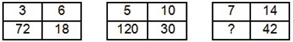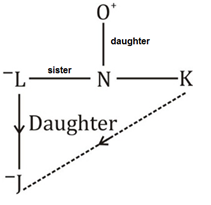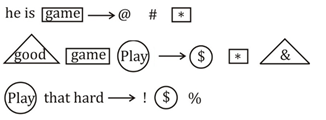# SSC Reasoning Ability Practice Questions (Day-46)

Dear Aspirants, The SSC Exams are going on at national level. Millions of candidates have eagerly prepared for the ongoing SSC exams such as CGL, CHSL, MTS, CPO, JE etc. SSC exams are usually conducted by the Staff Selection Commission to recruit the eligible candidates for various Central government departments. Mostly the selection process for all the SSC exams consists of the single or double phase of written examination based on the examination. Due to that, the written exam consisted of a certain syllabus and exam pattern. By following those regulations, the written exam has been conducted through an online mode. Based on the latest updated exam pattern and syllabus, here we have drafted the Reasoning Ability questions on a daily basis. So aspirants start your practice session with us and mould your skill in a perfect shape.

Start Quiz

1) If ‘P 3 Q’ means ‘P is daughter of Q’, ‘P 5 Q’ means ‘P is father of Q’, ‘P 7 Q’ means ‘P is mother of Q’ and ‘P 9 Q’ means ‘P is sister of Q’, then how is Jrelated to K in J 3 L 9 N 3 O 5 K?

(a) Mother

(b) Wife

(c) Niece

(d) Daughter

Direction (2-3): In the following question, select the word which cannot be formed using the letters of the given word.

2) RIVALRIES

(a) RIVAL

(b) RICE

(c) SEAL

(d) RISE

3) DICTIONARIES

(a) CATION

(b) SITE

(c) DICTATE

(d) TIRED

4) In a certain code language, “he is game” is written as “@#*”, “good game play” is written as “\$*&”and”playthat  hard” is written as “!\$%”.How is “good” written in that code language?

(a) &

(b) *

(c) \$

(d) @

5) In a certain code language, “CAB” is written as “6” and “LEG” is written as “6”. How is “MAP” written in that code language?

(a) 6

(b) 4

(c) 3

(d) 8

6) If “÷” denotes “subtracted from”, “+” denotes “multiplied by”, “–” denotes “added to” and “×” denotes “divided by”, then

19 + 2 ÷ 2 + 2 – 14 = ?

(a) 52

(b) 46

(c) 48

(d) 88

7) If “#” denotes “multiplied by”, “@” denotes “subtracted from”, “*” denotes “added to” and “%” denotes “divided by”, then which of the following equation must be true?

(a) 4 % 2 # 6 * 7 = 18

(b) 3 # 9 @ 4 # 6 = 4

(c) 5 # 6 % 3 @ 4 = 8

(d) 14 % 2 * 3 # 8 = 31

8) If 11 # 2 @ 6 = 78 and 15 # 4 @ 8 = 152, then 17 # 6 @ 7 = ?

(a) 161

(b) 143

(c) 221

(d) 157

9) If 2 = 0, 5 = 1 and 8 = 4, then 11 = ?

(a) 8

(b) 9

(c) 10

(d) 6

Direction (10): In the following question, select the number which can be placed at the sign of question mark (?) from the given alternatives.

10)(a) 84

(b) 136

(c) 144

(d) 168J is the niece of K

‘RICE’ ; There is no ‘C’ in the given word.

‘DICTATE’ ; There is no double ‘T’ in the given word.∴ Code for good → &

CAB = 3 + 1 + 2 = 6

LEG = 12 + 5 + 7 = 24 = 2 + 4 = 6

MAP = 13 + 1 + 16 = 30 = 3 + 0 = 3

19 + 2 ÷ 2 + 2 – 14

⇒ 19 × 2 – 2 × 2 + 14

⇒ 38 – 4 + 14

⇒ 48

14% 2 * 3 # 8 = 31

⇒ 14 ÷ 2 +  3 ×  8 = 31

⇒ 7 + 24 = 31

⇒ 31 = 31

(11 + 2) × 6 = 78

(15 + 4) × 8 = 152

(17 +  6) × 7 = 161

11 = 2 + 8 + 1

= 0 + 4 + 5

= 9

3 × 2 = 6 ;  6 × 3 = 18;   18 × 4 = 72

5 × 2 = 10;  10 × 3 = 30;  30 × 4 = 120

7 × 2 = 14;  14 × 3 = 42;  42 × 4 = 168

 Check Here to View SSC CGL / CHSL 2021 Reasoning Ability Questions Day – 45 Day – 44 Day – 43